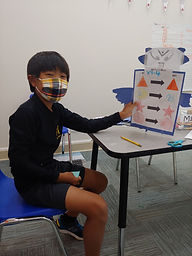## Ms. Mari

### Target 1​

###### Lesson Type:

Continuation

Algebra

:

Function

Use input and output data to determine a function equation.

###### 1:

Use a function equation to fill out a function table.

7th

###### Vocabulary:

FUNCTION, INPUT, OUTPUT

Activities:

- Finished creating function robot machines, in which they determined their own function rule and displayed four different inputs and outputs.

- Determined the rules for the other function robot machines created by classmates.### Home Exploration

###### Guiding Questions:## Absent Students:

### Target 2

:

###### 1:

Interpret the relationship between x and y values.

###### 2:

Identify the different representations of a function on a graph.

7th

###### Vocabulary:

FUNCTION, EQUATION, TABLE, GRAPH

Activities:

- Given a table of values, created a graph of the inputs and outputs.

- Determined the equation (y = ) that described the function.### Home Exploration

###### Guiding Questions:### Target 3

:

###### Vocabulary:

Activities:### Home Exploration